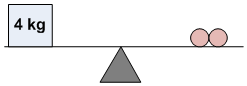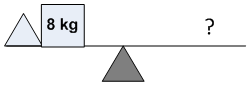### Sample Problem

How many balls you need to balance the last set-up?

IfAndThenballs

#### Solution

The mass of each ball: 4 kg ÷ 2 = 2 kg

The mass of 2 triangles: 2 kg x 6 = 12 kg

The mass of each triangle: 12 kg ÷ 2 = 6 kg

Then, the weight needed to be balanced: 6 kg + 8 kg = 14 kg

Thus, the balls needed: 14 kg ÷ 2 kg = 7